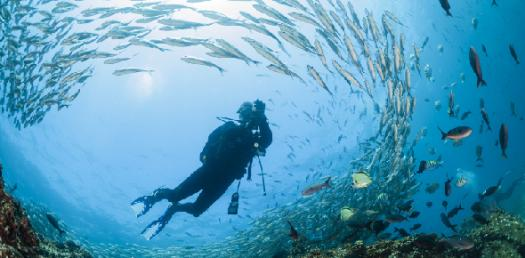# 1-a,2-a, 1-b,2-b 15.1 Assessment

15 Questions | Total Attempts: 251SettingsThis will cover concepts in Section 1 of Unit 15 Composition of Seawater.

Related Topics
• 1.
Take a look at this video.  Tell me how density, salinity and temperature are related.   Alike?   Different? http://www.sciencelearn.org.nz/Contexts/The-Ocean-in-Action/Sci-Media/Video/Why-salinity-and-temperature-are-measured
• 2.
The ocean's surface water temperature vaires with the amount of solar radiation received,  which is a direct function of ______________.
• A.

Latitude

• B.

Meredian

• C.

Longitude

• 3.
Take a look at this image.   Choose the BEST answer that describes this image.
• A.

The higher the temperature the higher the density

• B.

The lower the temperature the lower the density

• C.

The lower the temperature the higher the density

• 4.
Which of the following is NOT a process that DECREASES the salinity of seawater?
• A.

Runoff from land

• B.

Icebergs melting

• C.

Precipitation

• D.

Evaporation

• 5.
What is the salinity of seawater?
• A.

1.5 percent

• B.

3.5 percent

• C.

10 percent

• D.

25 percent

• 6.
In this layer of the ocean between 300 meters and 1000 meters, there is a rapid change of temperature with depth.
• A.

Surface zone

• B.

Thermocline

• C.

Abyssal zone

• 7.
What units are used to measure the salinity of ocean water?
• A.

Percent

• B.

Parts per billion

• C.

Parts per thousand

• D.

Parts per million

• 8.
Examine this image.     Sodium is the largest component of sea water
• A.

True

• B.

False

• 9.
Most of the salt in seawater is ____________  _________________   or common table salt.
• A.

Potassium chloride

• B.

Magnesium phosphate

• C.

Sodium chloride

• D.

Calcium carbonate

• 10.
All water, even rainwater contains dissolved chemicals that scientists call a_______.
• A.

Precipitates

• B.

Salts

• C.

Evaporates

• 11.
If the salt could be removed from the ocean there would be a layer _________ thick all over the surface of the Earth.
• A.

1500

• B.

1000

• C.

500

• D.

250

• 12.
Ocean water is a very weak solution of only 2 elements,  Sodium and Chlorine
• A.

True

• B.

False

• 13.
Rivers carry an estimated ___________ __________ tons of salts into the ocean annually.
• A.

1 million

• B.

4 billion

• C.

2 million

• D.

3 trillion

• 14.
The ocean is supplied with enough asaltsa to maintain its salinity for millions of years because of the
• A.

Carbon cycle.

• B.

Nitrogen cycle.

• C.

Hydrologic cycle.

• D.

Unicycle